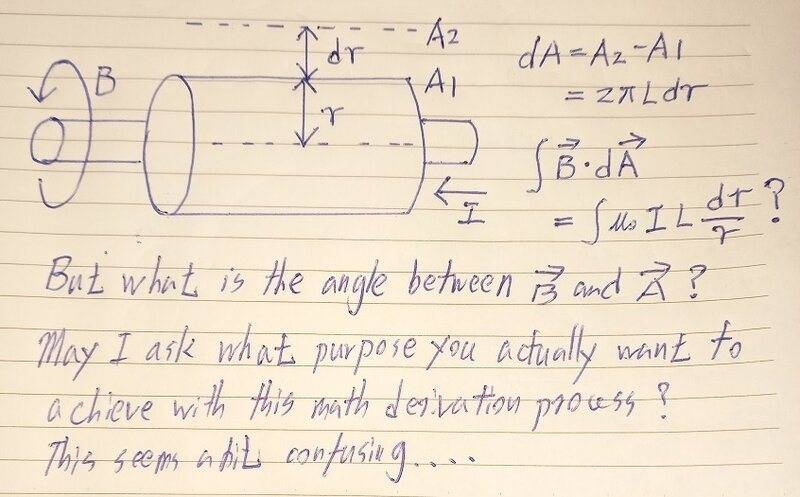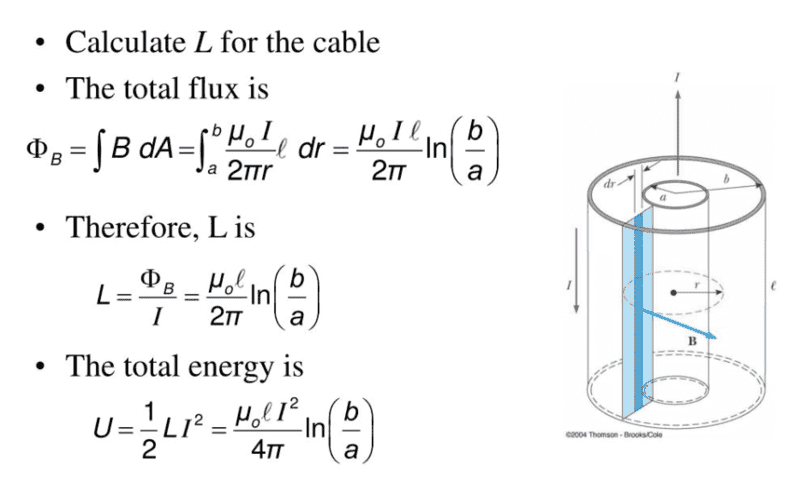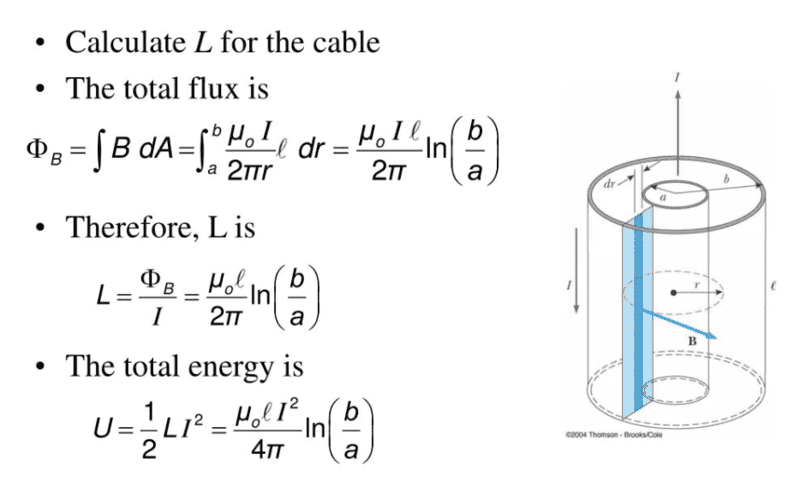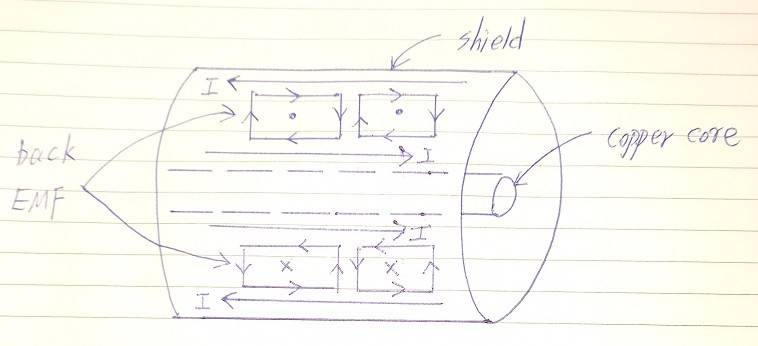# Derivation of the magnetic flux in coaxial cable

• I
• annamal
I am trying to get L the inductance and trying to figure out how they derived ##\phi_m## in the image attached. Mostly I don't get how they got dA = LdRThe flux denoted as ##\Phi_B## in that image is not the flux through the lateral surface of the cylinder, but the flux through the blue region. That blue region is not a closed surface so the flux of B can be non zero, no conflict with Gauss's law.The ##d\vec{A}## of the blue region is ##ldr## (it is the darker blue region of the picture) and points in the same direction as

#### annamal

The magnetic flux ##\phi_m = \int{BdA}##
The magnetic field of the coaxial cable B = ##\frac{I_{enc} \mu_0}{2\pi r}##
since surface area of a cylinder = ##2\pi rdr L, dA = 2\pi L dr## where L is the length of the coaxial cable
so ##\phi_m = \int{\frac{I_{enc} \mu_0}{2\pi r}2\pi L dr}##?

Last edited:
The integrand should be ##\vec{B}\cdot d\vec{A}##. Which direction does the B field point and which direction does the elementary area point?

•hutchphd and Delta2
And your expression for ##dA## is wrong. In what world do you do an integral over ##r## to calculate the curved surface area of a cylinder?

•hutchphd
Ibix said:
And your expression for ##dA## is wrong. In what world do you do an integral over ##r## to calculate the curved surface area of a cylinder?
isn't dA the surface area of the cylinder which should be ##A = 2\pi rL##; taking the derivative of that ##dA = 2\pi drL##

No. ##dA## is the elementary vector area - the area of a small rectangle. And again, why are you even thinking of differentiating with respect to ##r##, which is constant on the curved surface?

Cant be bothered to deal whether ##dA=2\pi Ldr## or not but I think it should be best if you try to answer the question in post #2. If you answer successfully you ll see that the dot product ##\vec{B}\cdot\vec{dA}## does not depend on the magnitude of ##\vec{dA}##.

Ibix said:
The integrand should be ##\vec{B}\cdot d\vec{A}##. Which direction does the B field point and which direction does the elementary area point?
vector dA is radial for the cylinder and the B field is perpendicular to that tangent to the circle of the cylinder

So ##\vec{B}\cdot d\vec{A}## = 0? That doesn't make sense because ##\phi_m## would equal 0?

You did not specify the surface through which you want to calculate the flux. Until you do this, there is no point to discuss the value of the flux. The "flux in coaxial cable" means nothing. The flux depends on a specific surface. What is this surface?

•Ibix and alan123hk
annamal said:
So ##\vec{B}\cdot d\vec{A}## = 0? That doesn't make sense because ##\phi_m## would equal 0?
It tells you that the flux through the surface of the conductor is zero, yes. This shouldn't be particularly surprising - remember that ##\int_S \vec{B}\cdot d\vec{A}=\int_V\vec{\nabla}\cdot\vec{B}dV## and if ##\vec{\nabla}\cdot\vec{B}\neq 0## we have a problem.

As @nasu points out, though, the flux through non-closed surfaces with different orientations may well be non-zero.

annamal said:
vector dA is radial for the cylinder and the B field is perpendicular to that tangent to the circle of the cylinder

So ##\vec{B}\cdot d\vec{A}## = 0? That doesn't make sense because ##\phi_m## would equal 0?
Remember Gauss's law for the magnetic field if S is any closed surface then $$\int_S\vec{B}\cdot d\vec{A}=0$$.

Of course what is missing from your calculation is the flux through the "caps" of the cylinder , but that can be easily shown to be zero as well for an azimuthal magnetic field.

annamal said:
The magnetic flux ϕm=∫BdA
The magnetic field of the coaxial cable B = Iencμ02πr
since surface area of a cylinder = 2πrdrL,dA=2πLdr where L is the length of the coaxial cable
so ϕm=∫Iencμ02πr2πLdr?

Could you explain further the purpose of the equation derivation?
I feel like there might be something misunderstood or incomprehensible.•Delta2
alan123hk said:
Could you explain further the purpose of the equation derivation?
I feel like there might be something misunderstood or incomprehensible.

I am trying to get L the inductance and trying to figure out how they derived ##\phi_m## in the image attached. Mostly I don't get how they got dA = LdR•alan123hk
The flux denoted as ##\Phi_B## in that image is not the flux through the lateral surface of the cylinder, but the flux through the blue region. That blue region is not a closed surface so the flux of B can be non zero, no conflict with Gauss's law.

•alan123hk and Ibix
The ##d\vec{A}## of the blue region is ##ldr## (it is the darker blue region of the picture) and points in the same direction as ##\vec{B}##.

annamal said:
I am trying to get L the inductance and trying to figure out how they derived ##\phi_m## in the image attached.
There's a lesson here. If you'd stated your whole question with context in your OP instead of waiting until alan123hk prompted you, we'd have been at this point 24 hours and 12 posts earlier.
annamal said:
Mostly I don't get how they got dA = LdR
The elementary area on a flat plane like the blue surface is ##dxdy##, the area of a rectangle of side ##dx## and ##dy##. So ##\int\mathrm{(something)}dA## in the case of a flat plane is ##\int\int\mathrm{(something)}dxdy##. In this case, though, your plane lies in the r-z plane - i.e. a small radial displacement is ##dr## and a small vertical displacement is ##dz##, so the elementary area is ##dA=drdz##. So the flux through the blue plane is $$\phi_B=\int_{r=a}^b\int_{z=0}^lB(r)drdz$$ (where I've ignored the dot product because ##B## is always normal to the blue plane). But note that the integrand does not depend on ##z##, so you can do the ##z## integral to get$$\phi_B=l\int_a^bB(r)dr$$which is the result in the image.

•nasu, alan123hk and Delta2
@annamal Thanks for the explanation, I misunderstood what you meant. Yes, that's the magnetic flux created in a coaxial cable. Then we can calculate the inductance of the coaxial cable based on the definition of inductance.Delta2 said:
The ##d\vec{A}## of the blue region is ##ldr## (it is the darker blue region of the picture) and points in the same direction as ##\vec{B}##.
Why isn't this region all the way around the cylinder of the inner coaxial cable?

annamal said:
Why isn't this region all the way around the cylinder of the inner coaxial cable?

I don't quite understand what you mean.

In any case, note that the magnetic field lines are directional and form a closed loop. Now we want to consider the total number of magnetic field lines generated by the current in the coaxial cable, which are formed by concentric circles around the center conductor, which of course are only included in the blue area of the diagram below.Last edited:
annamal said:
Why isn't this region all the way around the cylinder of the inner coaxial cable?
We choose this region because it has as boundaries the two conductors of the coaxial cable. From Faraday's law in integral form the EMF in the boundaries (that is in the conductors in this case )due to the time varying B, will be equal to the time derivative of the flux through this region. If we can bring the EMF in a form $$EMF=C\frac{dI}{dt}$$ where C might be functions of many things, except the time t, then C is by definition the desired L. This process is equivalent to bringing the flux in the form ##\Phi=CI## which is what is done in the picture you show.

Last edited:
•alan123hk and nasu
Delta2 said:
From Faraday's law in integral form the EMF in the boundaries (that is in the conductors in this case )due to the time varying B, will be equal to the time derivative of the flux through this region. If we can bring the EMF in a form
Yes, according to Faraday's law, a change in the magnetic field in this blue region will create a back EMF, and then this back EMF will try to impede the current flow in the copper core and shield of the coaxial cable. This is the same thing that happens when current flows in a coil.

•Delta2
I don't know what you are talking about. Are you looking at Ampere's circuital law in magnetostatics or at Faraday's Law? The former says (in the much simpler local form)
$$\vec{\nabla} \times \vec{B}=\mu_0 \vec{j}$$
and the latter
$$\vec{\nabla} \times \vec{E} = -\partial_t \vec{B}.$$

Delta2 said:
We choose this region because it has as boundaries the two conductors of the coaxial cable. From Faraday's law in integral form the EMF in the boundaries (that is in the conductors in this case )due to the time varying B, will be equal to the time derivative of the flux through this region. If we can bring the EMF in a form $$EMF=C\frac{dI}{dt}$$ where C might be functions of many things, except the time t, then C is by definition the desired L. This process is equivalent to bringing the flux in the form ##\Phi=CI## which is what is done in the picture you show.
What I mean is how come the area in ##\phi_m = BA## isn't all the way around the inner cylinder. That is the space between the outer and inner cylinders.

You are confusing surface (measured by area) with volume. The flux is defined through a surface and not in a volume. The space between the two cylinders is not a surface but a volume.

•alan123hk
The magnetic field always surrounds the inner cylinder, but you can find the total magnetic flux by simply selecting an area at any angle on the axis. This is because flux is defined through a surface, not volume.

But if you want to know the total energy stored in the magnetic field in space, you need to integrate over the volume.

vanhees71 said:
I don't know what you are talking about. Are you looking at Ampere's circuital law in magnetostatics or at Faraday's Law? The former says (in the much simpler local form)
I mean according to Faraday's law, the back EMF created by the magnetic flux impedes the original current flow in the copper core and shield, which is what causes the inductance of the coaxial cable.annamal said:
What I mean is how come the area in ##\phi_m = BA## isn't all the way around the inner cylinder. That is the space between the outer and inner cylinders.
You just can't take as surface the lateral surface of any cylinder with axis the axis of the cable. That is because such a surface doesn't have as boundary the inner and outer conductor, so if you calculate the flux and EMF through such a surface (which EMF would be zero for such a surface), is isn't the EMF generated in the conductors.

P.S I am not sure if we can define the boundary of the lateral surface of a cylinder. Not it isn't the outer or the inner conductor of the cable, as you probably might thinking.

Faraday's Law reads (in integral form assuming a surface and boundary at rest in the reference frame under consideration)
$$\int_{\partial A} \mathrm{d} \vec{r} \cdot \vec{E}=-\frac{\mathrm{d}}{\mathrm{d} t} \int_A \mathrm{d}^2 \vec{f} \cdot \vec{B},$$
i.e., you need to specify an oriented surface with a boundary (a closed curved) directed relative to the orientation of the surface normal vectors according to the right-hand rule. Otherwise you can't apply Faraday's rule. I'm still puzzled about the question, what you want to achieve.

•Delta2